Next: Symmetry Restraints Up: User Defined Forces Previous: Moving Constraints   Contents   Index

## Rotating Constraints

The constraints parameters are specified in the same manner as for usual (static) harmonic constraints. The reference positions of all constrained atoms are then rotated with a given angular velocity about a given axis. If the force constant of the constraints is sufficiently large, the constrained atoms will follow their reference positions.

A rotation matrix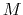about the axis unit vector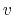is calculated every timestep for the angle of rotation corresponding to the current timestep. angle =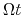, where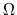is the angular velocity of rotation.

From now on, all quantities are 3D vectors, except the matrixand the force constant.

The current reference position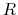is calculated from the initial reference position(at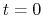),, whereis the pivot point.

Coordinates of point N can be found as. Normal from the atom pos to the axis is, similarly, normalThe force is, as usual,; This is the force applied to the atom in NAMD (see below). NAMD does not know anything about the torque applied. However, the torque applied to the atom can be calculated as a vector product torque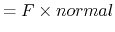Finally, the torque applied to the atom with respect to the axis is the projection of the torque on the axis, i.e.,If there are atoms that have to be constrained, but not moved, this implementation is not suitable, because it will move all reference positions.

Only one of the moving and rotating constraints can be used at a time.

Using very soft springs for rotating constraints leads to the system lagging behind the reference positions, and then the force is applied along a direction different from the "ideal" direction along the circular path.

Pulling on N atoms at the same time with a spring of stiffness K amounts to pulling on the whole system by a spring of stiffness NK, so the overall behavior of the system is as if you are pulling with a very stiff spring if N is large.

In both moving and rotating constraints the force constant that you specify in the constraints pdb file is multiplied by 2 for the force calculation, i.e., if you specified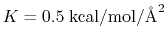in the pdb file, the force actually calculated is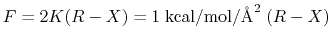. SMD feature of namd2 does the calculation without multiplication of the force constant specified in the config file by 2.

• rotConstraints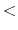Are rotating constraints activeAcceptable Values: on or off
Default Value: off
Description: Should rotating restraints be applied to the system. If set to on, then rotConsAxis, rotConsPivot and rotConsVel must be defined. May not be used with movingConstraints.

• rotConsAxisAxis of rotationAcceptable Values: vector (may be unnormalized)
Description: Axis of rotation. Can be any vector. It gets normalized before use. If the vector is 0, no rotation will be performed, but the calculations will still be done.

• rotConsPivotPivot point of rotationAcceptable Values: position in Å
Description: Pivot point of rotation. The rotation axis vector only gives the direction of the axis. Pivot point places the axis in space, so that the axis goes through the pivot point.

• rotConsVelAngular velocity of rotationAcceptable Values: rate in degrees per timestep
Description: Angular velocity of rotation, degrees/timestep.Next: Symmetry Restraints Up: User Defined Forces Previous: Moving Constraints   Contents   Index
http://www.ks.uiuc.edu/Research/namd/